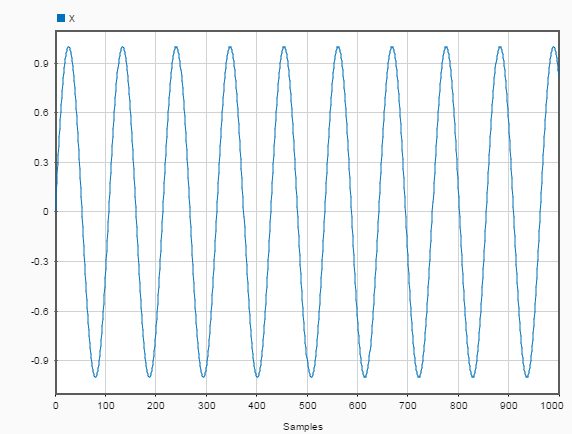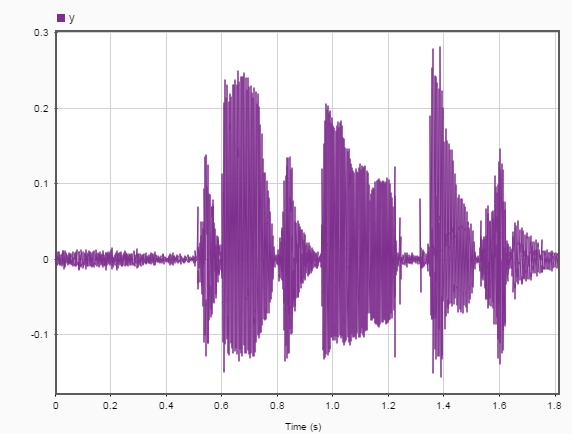## Edit Sample Rate and Other Time Information

You can add and edit the time information in the Signal Analyzer app for any signal that is not a `timetable` or a `timeseries` object. Select one or more signals with no inherent time information in the Signal table and on the Analyzer tab, click .

Note

Select a signal in the Signal table by clicking its Name column. The complete row is highlighted, indicating that the signal is selected. The check box next to the name of the signal indicates whether or not the signal is plotted in the active display.

In the Time Values dialog box, select a Time Specification option.

Time Specification OptionDescription
`Work in Samples` (default)

This option enables you to explore signals without the need to specify a sample rate or a sample time. It is equivalent to plotting the signal in MATLAB® without x-axis information.

`Sample Rate and Start Time`

Use this option when you know the rate at which the signal has been sampled. Specify the sample rate and the instant corresponding to the first sample.

The Sample Rate can be expressed in Hz, kHz, MHz, or GHz.

The Start Time can be expressed in seconds, years, days, hours, minutes, milliseconds, microseconds, or nanoseconds.

Set the sample rate so that the signal is plotted in units of time on a display.

`Sample Time and Start Time`

Use this option when you know the time interval between samples. Specify the sample time and the instant corresponding to the first sample.

The Sample Time and Start Time each can be expressed in seconds, years, days, hours, minutes, milliseconds, microseconds, or nanoseconds.

Set the sample time so that the signal is plotted in units of time on a display.

`Time Values`

Use this option when you know the time value corresponding to each sample. Specify the time values using a MATLAB expression or the name of a variable in the MATLAB workspace.

The Time Values can be stored in a numeric vector with real time values expressed in seconds. The values must be unique and cannot be `NaN`, but they need not be uniformly spaced. The vector must have the same length as the signal.

The time values can also be stored in a `duration` array. The values must be unique and cannot be `NaN`, but they need not be uniformly spaced. The array must have the same length as the signal.

The time values can also be entered as a MATLAB expression. The expression must specify an array with the same length as the signal. The values must be unique and cannot be `NaN`, but they need not be uniformly spaced. Valid examples include:

• `(0:length(s)-1)'/Fs`, where `s` is the signal and `Fs` is a scalar in the workspace representing a sample rate.

• `linspace(2,2.5,length(s))'`, where `s` is the signal.

• `minutes(0:15)'`, equivalent to taking measurements every minute for 15 minutes.

• `[0:10 20:30]`, equivalent to taking two sets of measurements at 1 Hz with a long pause between the sets.

In all cases, the app derives a sample rate from the time values and displays it in the Time column of the Signal table. An asterisk preceding the sample rate indicates that the signal is nonuniformly sampled.

Note

Signals with no time information are plotted in units of samples on the x-axis. Signals with time information are plotted in units of time on the x-axis. To plot several signals on the same display, ensure that they all have time information or are all in samples. Otherwise, you get a warning:Signal with x-axis in samples Signal with x-axis in time

• If a signal has missing or duplicate time points, you can fix it using the tips in Clean Timetable with Missing, Duplicate, or Nonuniform Times.

• If a signal is nonuniformly sampled, then Signal Analyzer interpolates the signal to a uniform grid to compute spectral estimates. The app uses linear interpolation and assumes a sample time equal to the median difference between adjacent time points. The derived sample rate in the Signal table has an asterisk to indicate that the signal is nonuniformly sampled.

Note

The interpolation is used only to compute spectral estimates. Time plots are not resampled.

For a nonuniformly sampled signal to be supported, the median time interval and the mean time interval must obey

• Filtering and scalogram view do not support nonuniformly sampled signals.

• The app does not support adding time information to `labeledSignalSet` objects or editing the time information of `labeledSignalSet` objects.